## Instructions

The goal of this project is prredicting the age of abalone from physical measurements. The age of abalone is determined by cutting the shell through the cone, staining it, and counting the number of rings through a microscope – a boring and time-consuming task. Other measurements, which are easier to obtain, are used to predict the age. Further information, such as weather patterns and location (hence food availability) may be required to solve the problem.

## Libraries

``````  library(ggplot2)
library(caret)``````
``## Warning: package 'caret' was built under R version 3.3.3``
``## Warning: package 'lattice' was built under R version 3.3.3``

Read csv in a data frame and formatting data

``````    # Read CSV data

# Clean NAs and empty values from dataset
data_abalone[data_abalone==""] <- NA
data_abalone <- na.omit(data_abalone)

# Create a column name from abalone.txt to get a description of each record value
colnames(data_abalone) <- c('Sex', 'Length', 'Diameter', 'Height', 'WholeWeight', 'ShuckedWeight',
'VisceraWeight', 'ShellWeight', 'Rings')

# Get a summary of data
summary(data_abalone)``````
``````##  Sex          Length         Diameter          Height
##  F:1307   Min.   :0.075   Min.   :0.0550   Min.   :0.0000
##  I:1342   1st Qu.:0.450   1st Qu.:0.3500   1st Qu.:0.1150
##  M:1528   Median :0.545   Median :0.4250   Median :0.1400
##           Mean   :0.524   Mean   :0.4079   Mean   :0.1395
##           3rd Qu.:0.615   3rd Qu.:0.4800   3rd Qu.:0.1650
##           Max.   :0.815   Max.   :0.6500   Max.   :1.1300
##   WholeWeight     ShuckedWeight    VisceraWeight     ShellWeight
##  Min.   :0.0020   Min.   :0.0010   Min.   :0.0005   Min.   :0.0015
##  1st Qu.:0.4415   1st Qu.:0.1860   1st Qu.:0.0935   1st Qu.:0.1300
##  Median :0.7995   Median :0.3360   Median :0.1710   Median :0.2340
##  Mean   :0.8287   Mean   :0.3594   Mean   :0.1806   Mean   :0.2388
##  3rd Qu.:1.1530   3rd Qu.:0.5020   3rd Qu.:0.2530   3rd Qu.:0.3290
##  Max.   :2.8255   Max.   :1.4880   Max.   :0.7600   Max.   :1.0050
##      Rings
##  Min.   : 1.000
##  1st Qu.: 8.000
##  Median : 9.000
##  Mean   : 9.934
##  3rd Qu.:11.000
##  Max.   :29.000``````
``````    # Get type of data
sapply(data_abalone, class)``````
``````##           Sex        Length      Diameter        Height   WholeWeight
##      "factor"     "numeric"     "numeric"     "numeric"     "numeric"
## ShuckedWeight VisceraWeight   ShellWeight         Rings
##     "numeric"     "numeric"     "numeric"     "integer"``````

## Plotting data

We will plot some figures and summaries(histograms from variables),…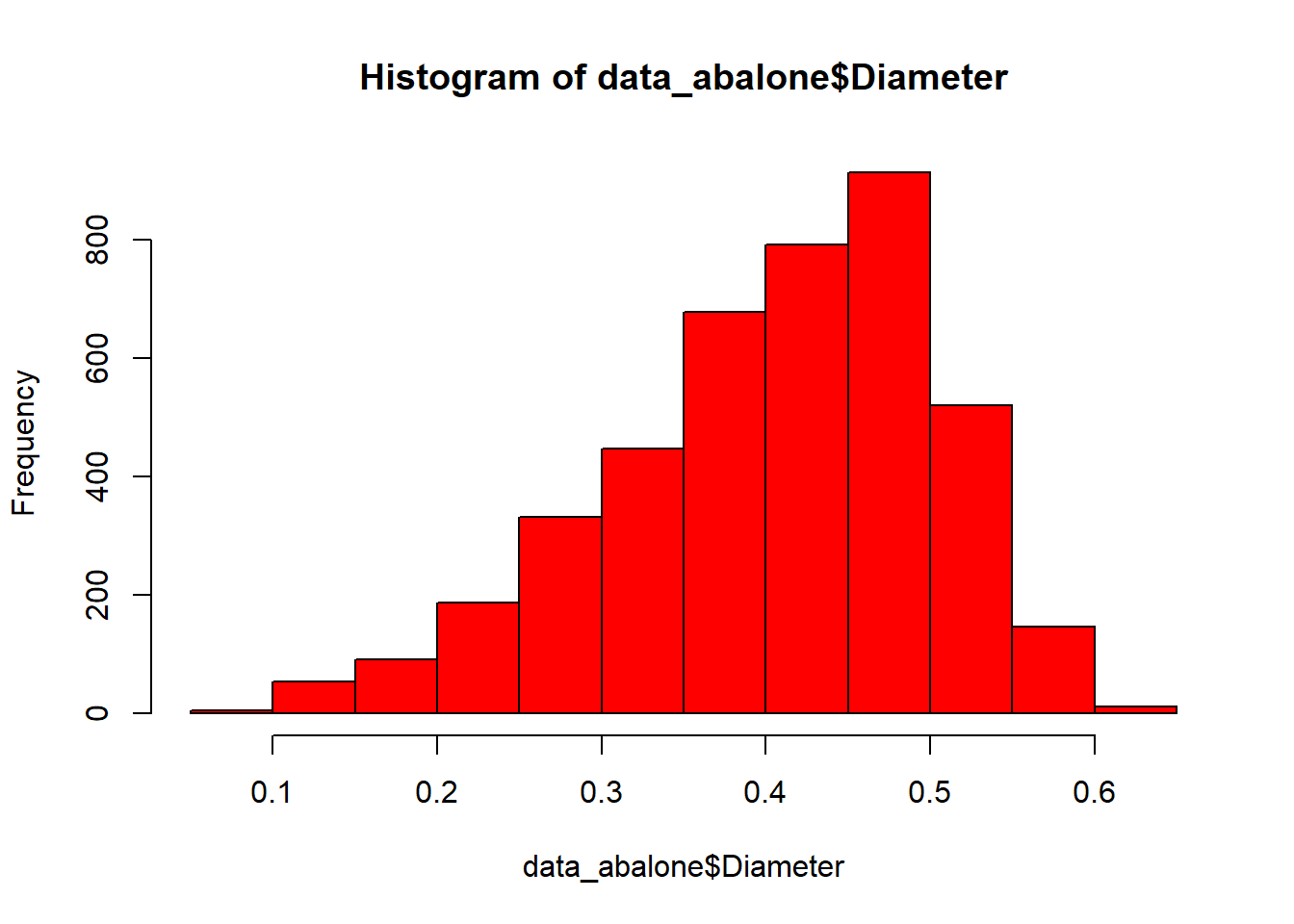``````##    Min. 1st Qu.  Median    Mean 3rd Qu.    Max.
##  0.0550  0.3500  0.4250  0.4079  0.4800  0.6500``````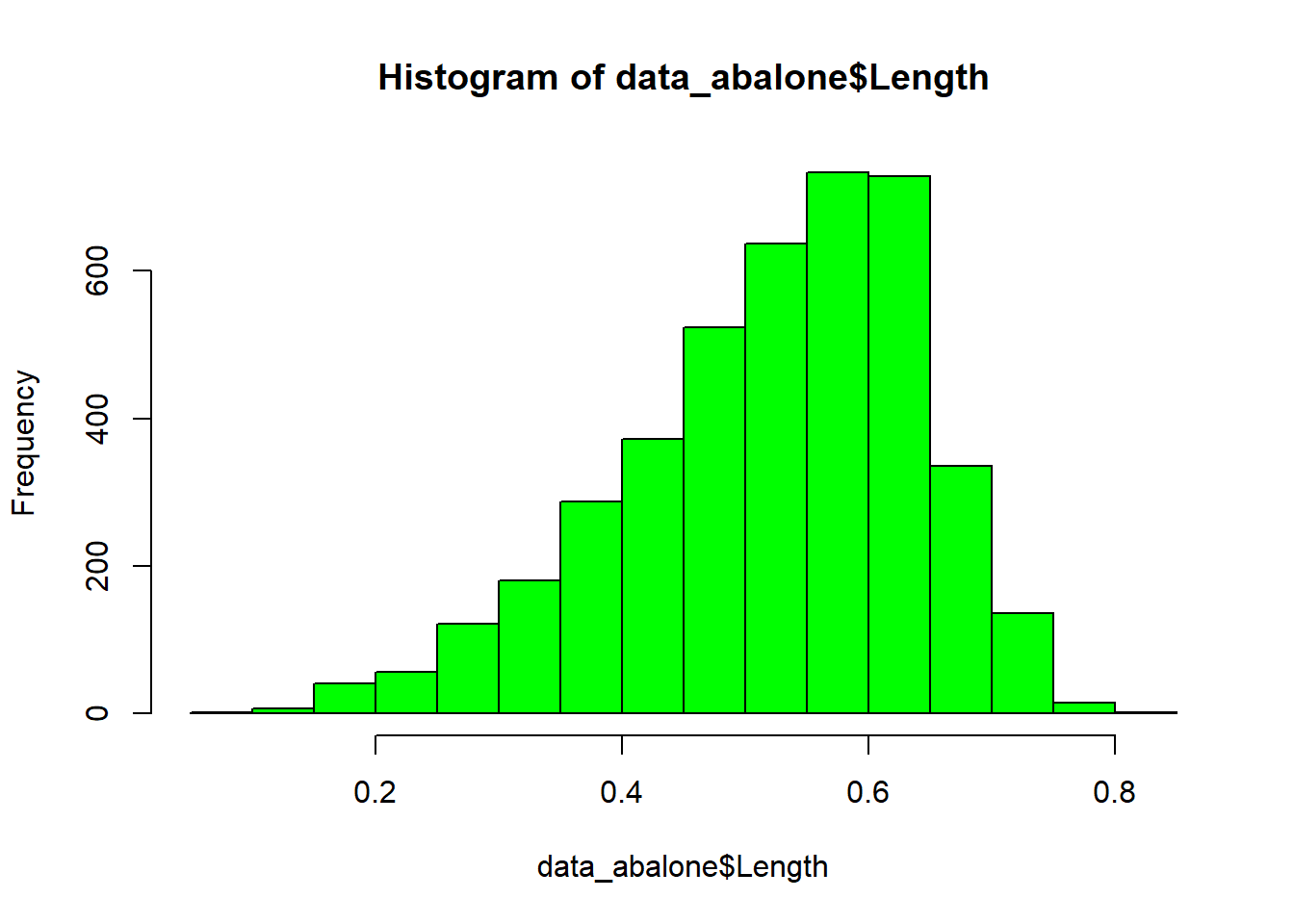``````##    Min. 1st Qu.  Median    Mean 3rd Qu.    Max.
##   0.075   0.450   0.545   0.524   0.615   0.815``````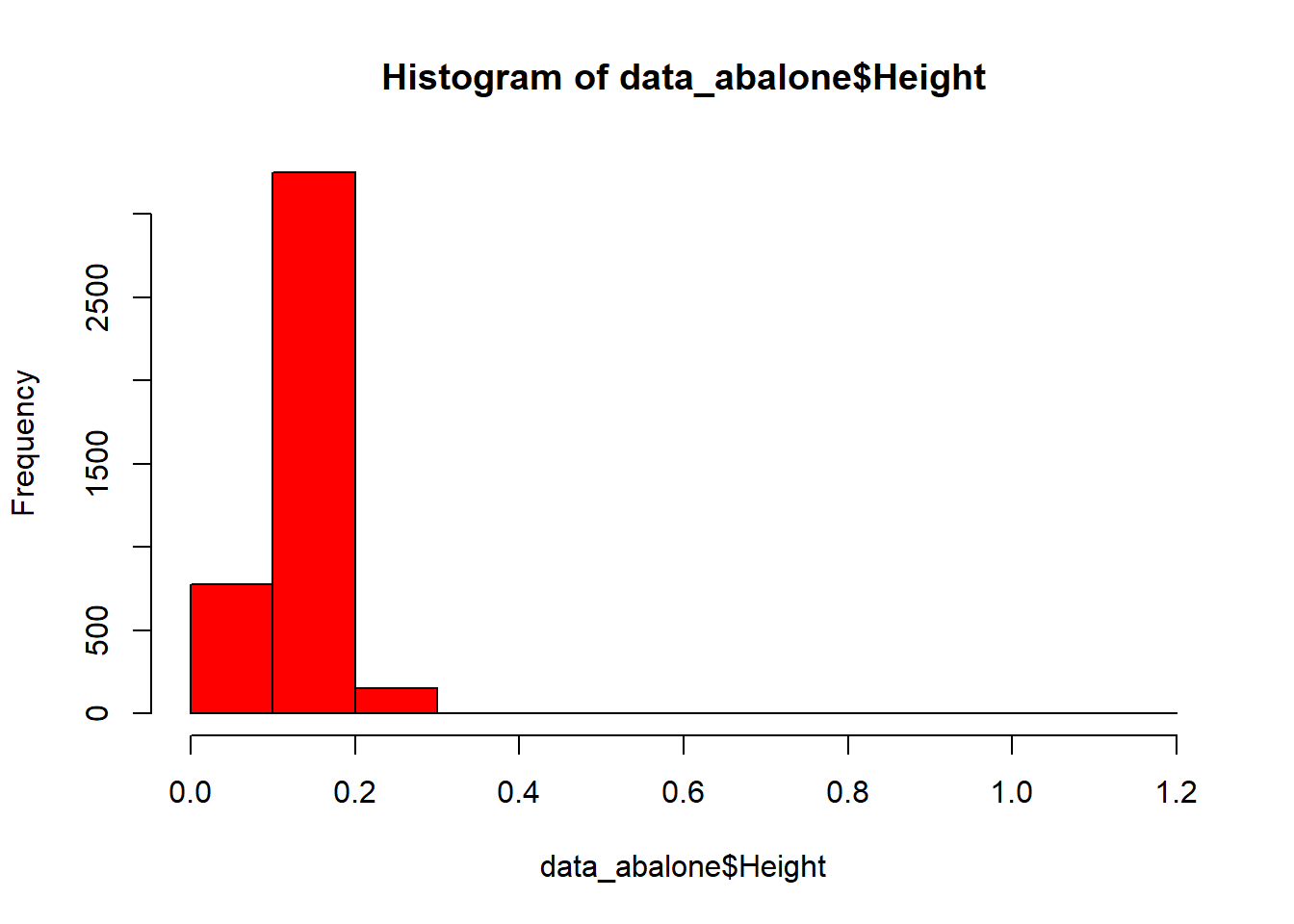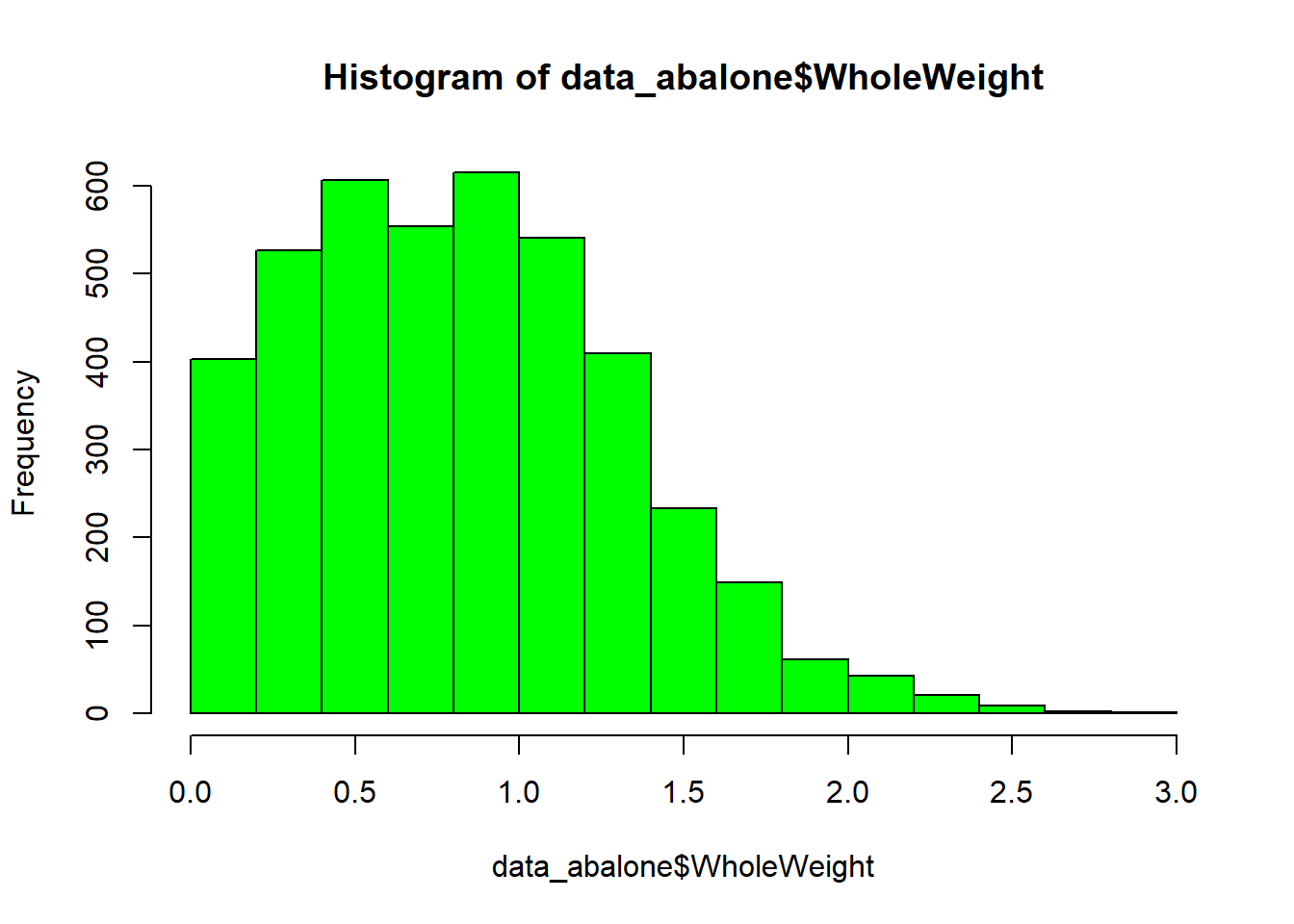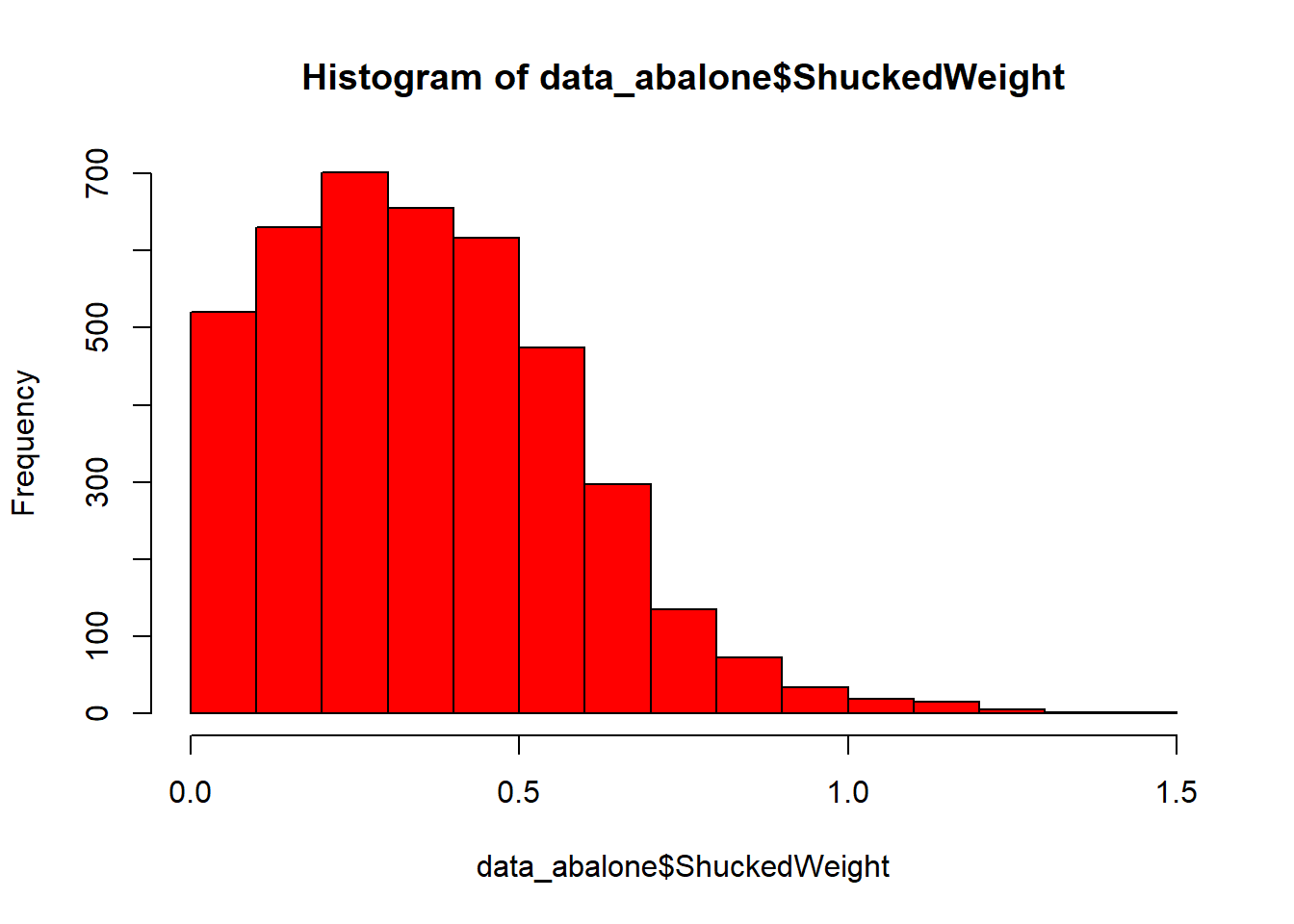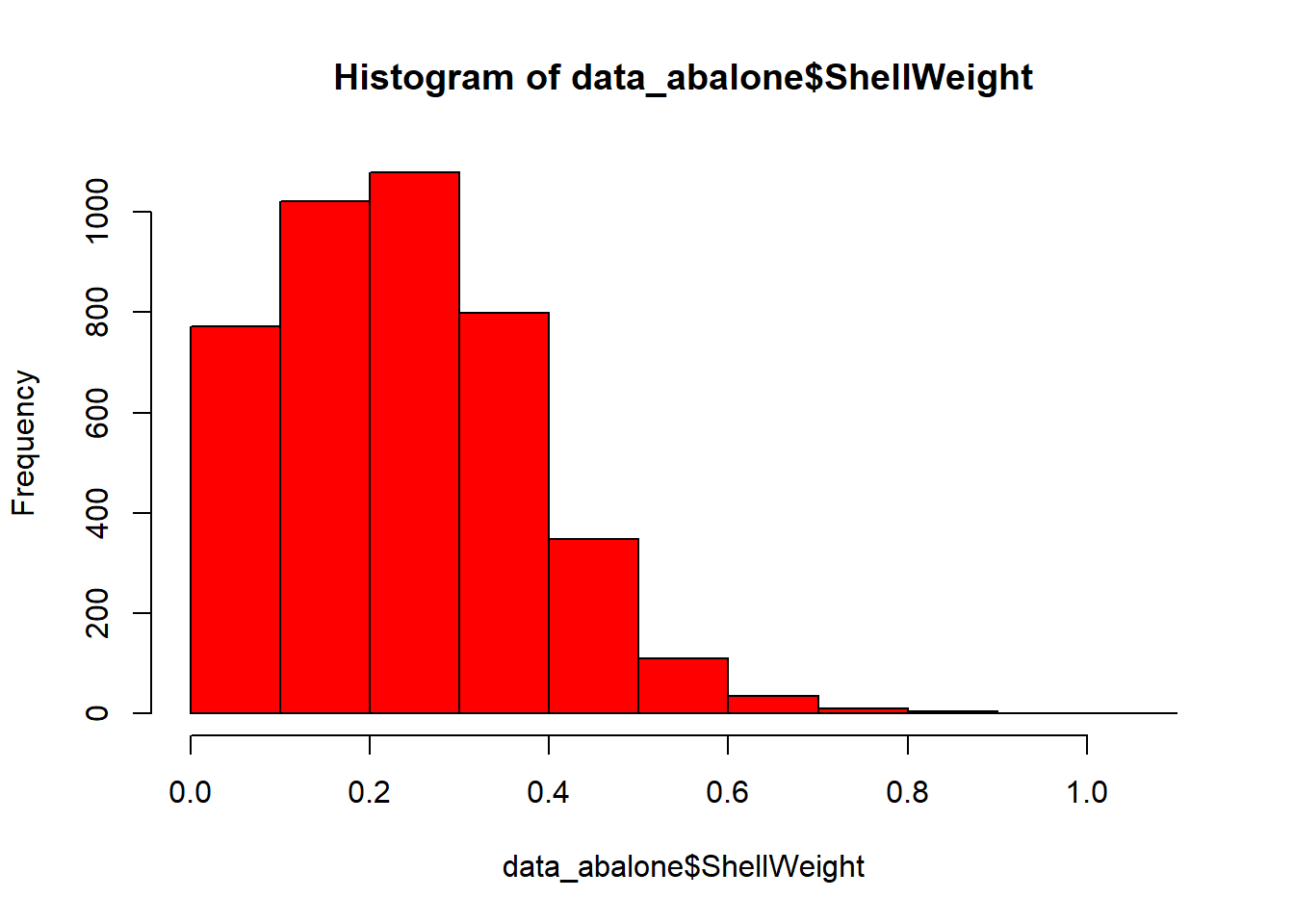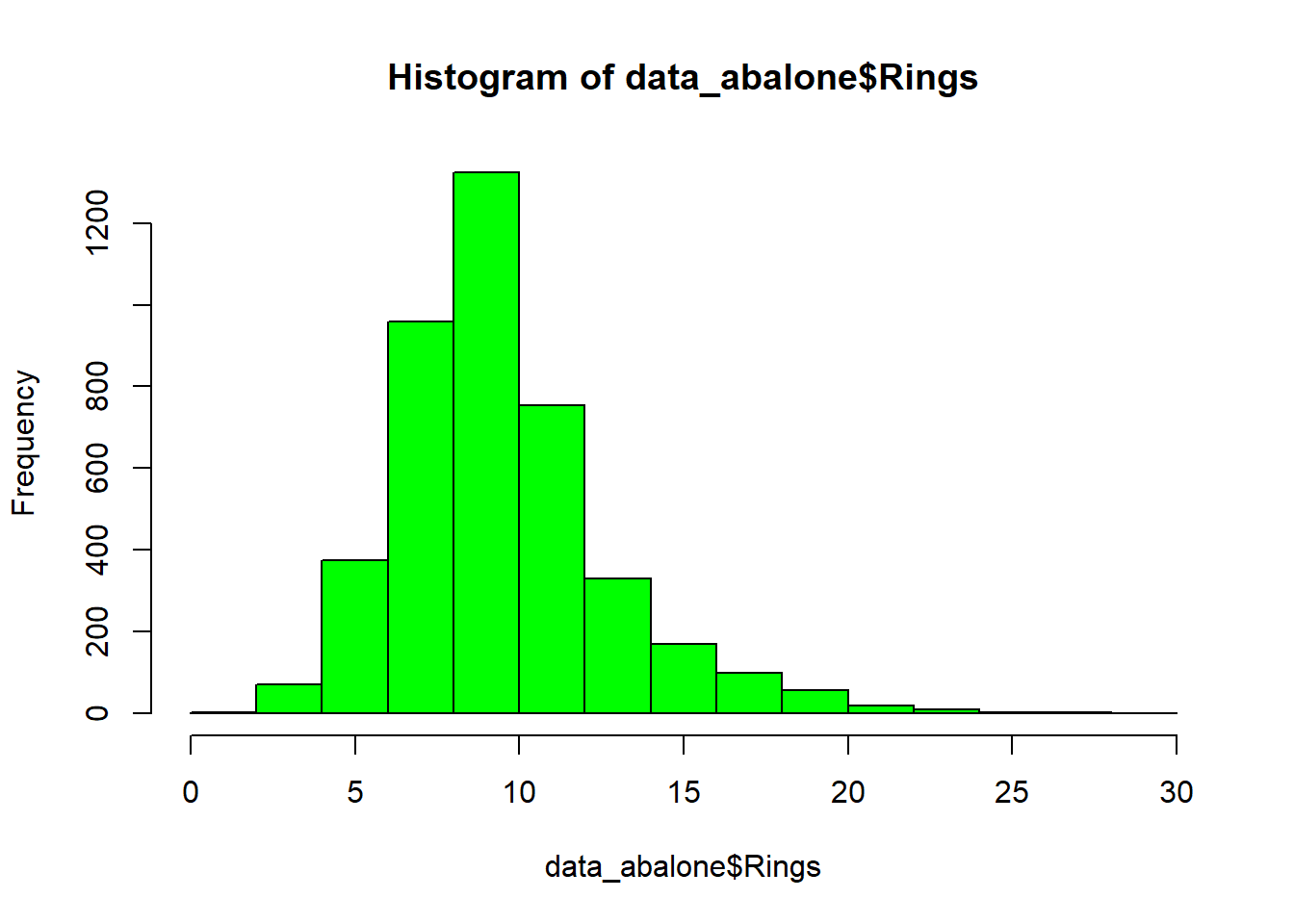``````##    Min. 1st Qu.  Median    Mean 3rd Qu.    Max.
##   1.000   8.000   9.000   9.934  11.000  29.000``````

## Predicting Age

### Correlation analysis

We will look correlation between data variables and rings in order to get some kind of linear relations which allow as to predict age(age=rings+1.5)

``    cor(data_abalone\$Length, data_abalone\$Rings, use="complete.obs", method="pearson") ``
``##  0.5567196``
``    cor(data_abalone\$Diameter, data_abalone\$Rings, use="complete.obs", method="pearson") ``
``##  0.5746599``
``    cor(data_abalone\$Height, data_abalone\$Rings, use="complete.obs", method="pearson")``
``##  0.5574673``
``    cor(data_abalone\$WholeWeight, data_abalone\$Rings, use="complete.obs", method="pearson")``
``##  0.5403897``
``    cor(data_abalone\$ShuckedWeight, data_abalone\$Rings, use="complete.obs", method="pearson")``
``##  0.4208837``
``    cor(data_abalone\$VisceraWeight, data_abalone\$Rings, use="complete.obs", method="pearson")``
``##  0.5038192``
``    cor(data_abalone\$ShellWeight, data_abalone\$Rings, use="complete.obs", method="pearson")``
``##  0.627574``
``````    # Looking through variables seems to be a correlation between Diameter (or Length or Height) with Rings
# We will explore this posibility``````

### Plotting Age Linear Models

According to correlation results more correlation is between ShellWeight and Rings and Length and Rings

``    qplot(Length, Rings, data=data_abalone, color=Sex) + geom_point() + geom_smooth(method="lm")``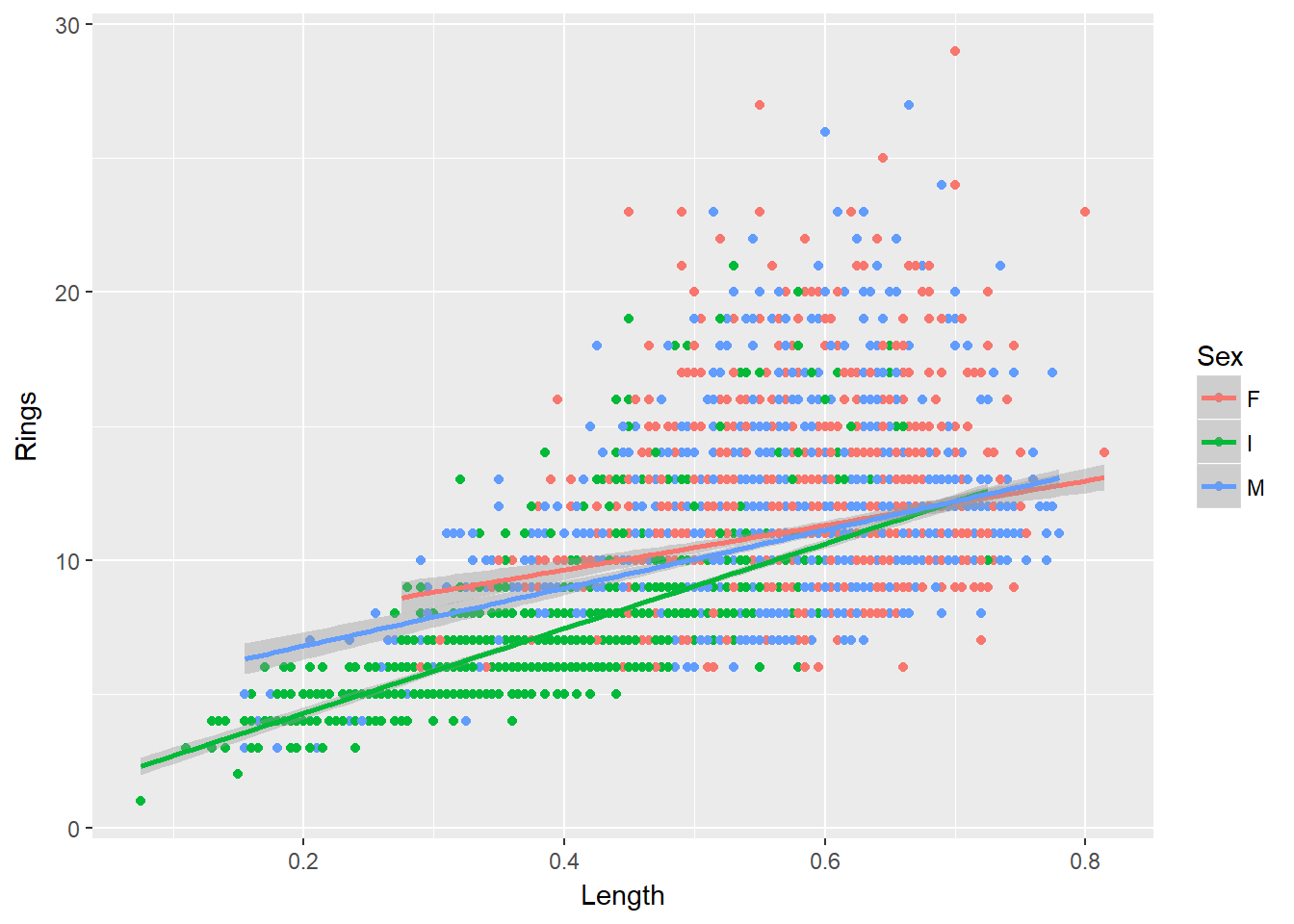``    qplot(ShellWeight, Rings, data=data_abalone, color=Sex) + geom_point() + geom_smooth(method="lm")``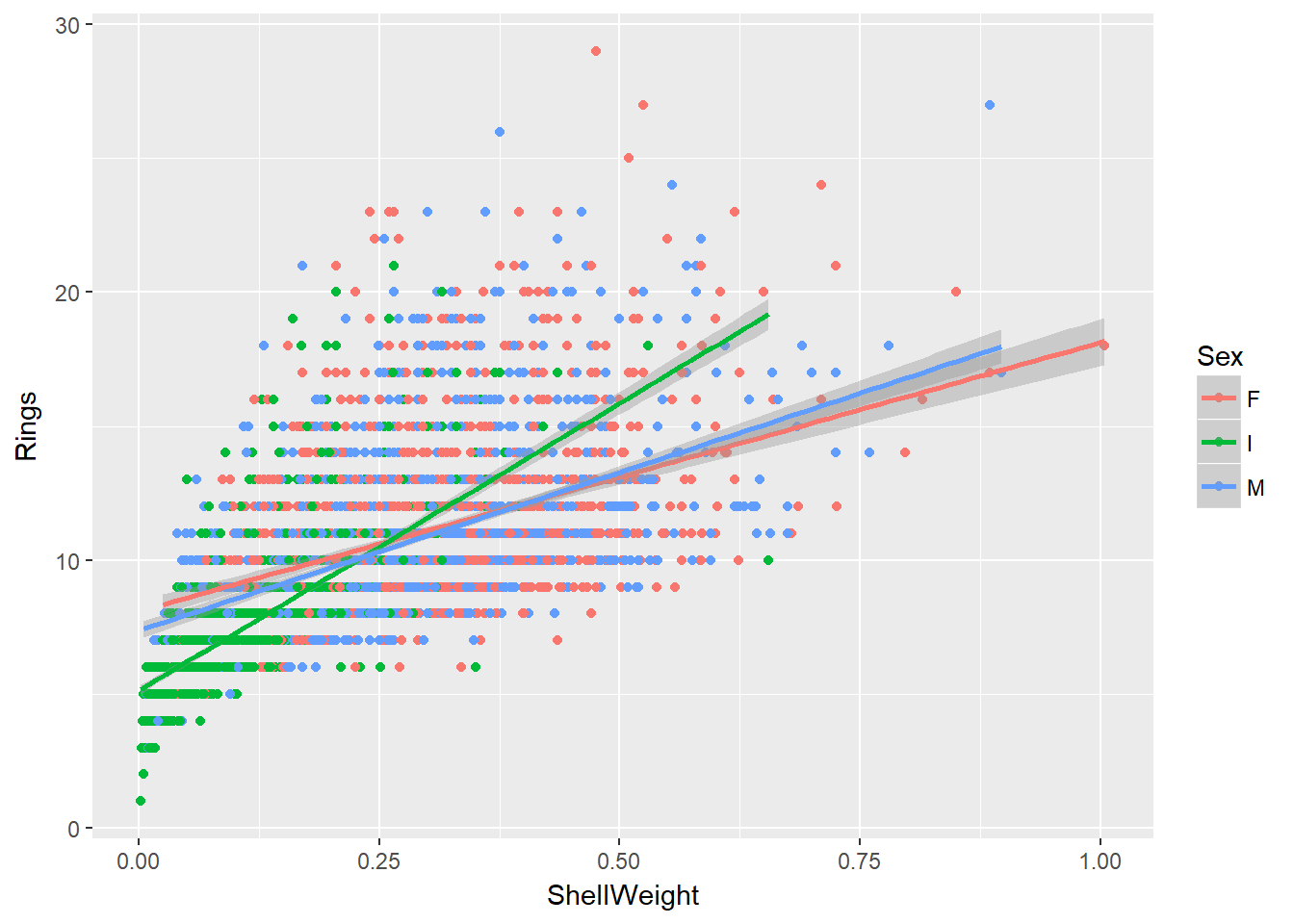### Comparison linear models

We will compare some models in order to select the best

``````    modelFitAll <- lm(Rings ~ ., data = data_abalone)
modelFitMultiple <- lm(Rings ~ ShellWeight + Length, data = data_abalone)
modelFitShellWeight <- lm(Rings ~ ShellWeight, data = data_abalone)
plot(resid(modelFitShellWeight), col="Blue")``````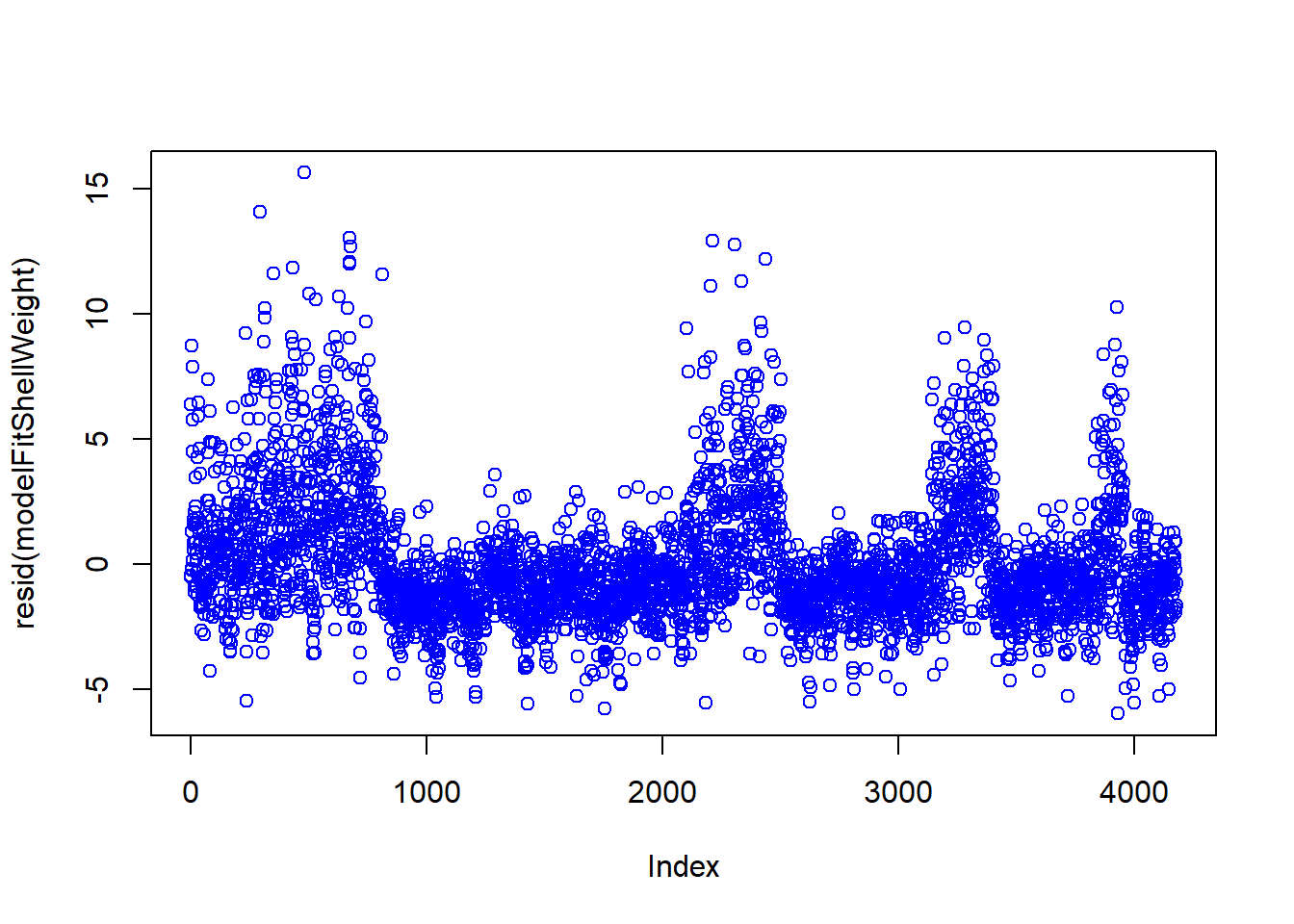``    anova(modelFitMultiple, modelFitShellWeight, test="Chisq")``
``````## Analysis of Variance Table
##
## Model 1: Rings ~ ShellWeight + Length
## Model 2: Rings ~ ShellWeight
##   Res.Df   RSS Df Sum of Sq Pr(>Chi)
## 1   4174 26304
## 2   4175 26313 -1   -9.9104   0.2098``````
``    anova(modelFitAll, modelFitShellWeight, test="Chisq")``
``````## Analysis of Variance Table
##
## Model 1: Rings ~ Sex + Length + Diameter + Height + WholeWeight + ShuckedWeight +
##     VisceraWeight + ShellWeight
## Model 2: Rings ~ ShellWeight
##   Res.Df   RSS Df Sum of Sq  Pr(>Chi)
## 1   4167 20061
## 2   4175 26313 -8   -6252.7 < 2.2e-16 ***
## ---
## Signif. codes:  0 '***' 0.001 '**' 0.01 '*' 0.05 '.' 0.1 ' ' 1``````
``````    #Seems than better model is taking into account all variables to predict Rings
summary(modelFitAll)``````
``````##
## Call:
## lm(formula = Rings ~ ., data = data_abalone)
##
## Residuals:
##      Min       1Q   Median       3Q      Max
## -10.4800  -1.3053  -0.3428   0.8600  13.9426
##
## Coefficients:
##                Estimate Std. Error t value Pr(>|t|)
## (Intercept)     3.89464    0.29157  13.358  < 2e-16 ***
## SexI           -0.82488    0.10240  -8.056 1.02e-15 ***
## SexM            0.05772    0.08335   0.692    0.489
## Length         -0.45834    1.80912  -0.253    0.800
## Diameter       11.07510    2.22728   4.972 6.88e-07 ***
## Height         10.76154    1.53620   7.005 2.86e-12 ***
## WholeWeight     8.97544    0.72540  12.373  < 2e-16 ***
## ShuckedWeight -19.78687    0.81735 -24.209  < 2e-16 ***
## VisceraWeight -10.58183    1.29375  -8.179 3.76e-16 ***
## ShellWeight     8.74181    1.12473   7.772 9.64e-15 ***
## ---
## Signif. codes:  0 '***' 0.001 '**' 0.01 '*' 0.05 '.' 0.1 ' ' 1
##
## Residual standard error: 2.194 on 4167 degrees of freedom
## Multiple R-squared:  0.5379, Adjusted R-squared:  0.5369
## F-statistic: 538.9 on 9 and 4167 DF,  p-value: < 2.2e-16``````
``    plot(modelFitAll)``